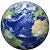Write a Python program to calculate the length of a string,

# Python program to calculate the length of a string.

def string_length(str1):

count = 0

for char in str1:

count += 1

return count

print(string_length(‘Python Program’))

--

--